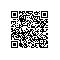# PHP版本繁简体,UTF-8和Unicode互转的函数:GB2312Big5，GB2312UTF-8，Big5UTF-8等<?phpfunction dirtree($path="."{include_once 'language.inc';$d = dir($path);while(false !== ($v = $d->read())){if($v == "." || $v == ".."continue$file = $d->path."/".$vif(is_dir($file)){//echo "$v";dirtree($file);}elseecho$file."\n";//rename($file,str_replace('zh_tw','zh_cn',$file));//$filename='zh_cn.lang.php';$fd=fopen($file,'r');$contents = fread($fd, filesize($file));$contents=u2b($contents);$contents=b2g($contents);//echo $contents;$contents=str_replace('日','日历',$contents);$contents=g2u($contents);$contents=str_replace('Trade chinese language pack created by METO, Inc. http://www.meto.com.tw','Simple chinese language pack created by Steed Soft Technology, Inc. http://www.steedsoft.com',$contents);fclose($fd);$fd=fopen(str_replace('zh_tw/','zh_cn/',str_replace('zh_tw','zh_cn',$file)),'w');fwrite($fd,$contents);fclose($fd);//rename($file,str_replace('zh_tw','zh_cn',$file));}$d->close();//echo " ";}dirtree(
"zh_tw");?>

P.S使用钉钉扫一扫加入圈子
+ 订阅